# GPT2 Transformer Trained onWebText Data

Generate text in English and represent text as a sequence of vectors

Released in 2019, this model improves and scales up its predecessor model. It has a richer vocabulary and uses BPE tokenization on UTF-8 byte sequences and additional normalization at the end of all of the transformer blocks.

Number of models: 3

## Training Set Information

• Preliminary version of the OpenWebText dataset, consisting of 40 GB of text scraped from webpages that have been curated by humans.

## Performance

• Accuracy of the models for various datasets: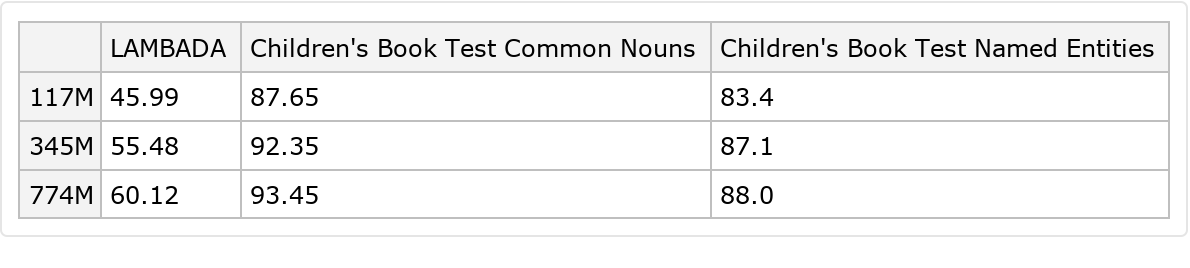Bits-per-character of the models for various datasets: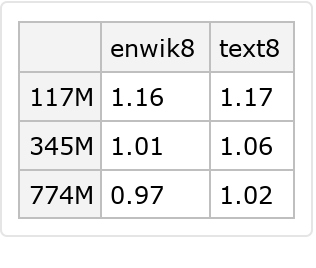Perplexity of the models for various datasets: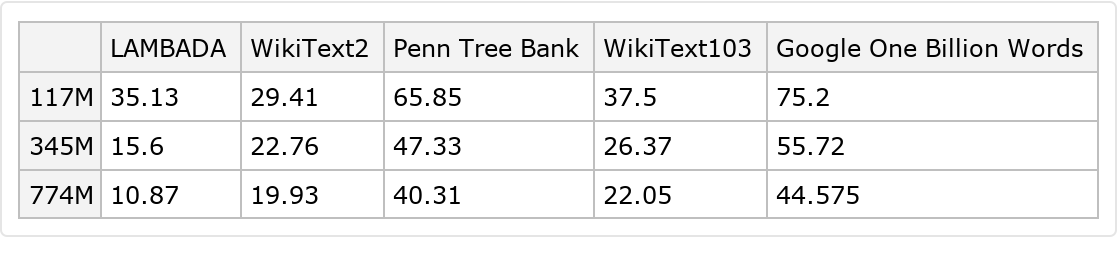## Examples

### Resource retrieval

Get the pre-trained net:

 In:=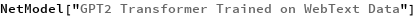Out=### NetModel parameters

This model consists of a family of individual nets, each identified by a specific parameter combination. Inspect the available parameters:

 In:=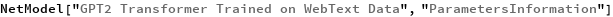Out=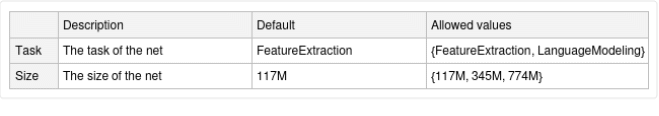Pick a non-default net by specifying the parameters:

 In:=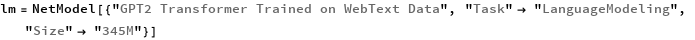Out=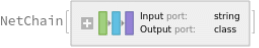Pick a non-default uninitialized net:

 In:=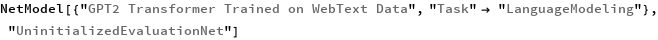Out=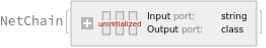### Basic usage

Given a piece of text, the GPT-2 net produces a sequence of feature vectors of size 768, which corresponds to the sequence of input words or subwords:

 In:=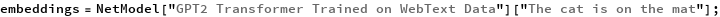Obtain dimensions of the embeddings:

 In:=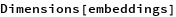Out=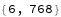Visualize the embeddings:

 In:=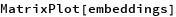Out=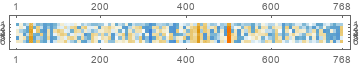### Transformer architecture

The input string is first normalized and then tokenized, or split into words or subwords. This two-step process is accomplished using the NetEncoder "Function":

 In:=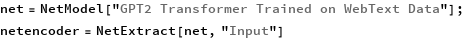Out=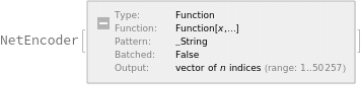The tokenization step is performed using the NetEncoder "BPESubwordTokens" and can be extracted using the following steps:

 In:=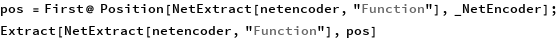Out=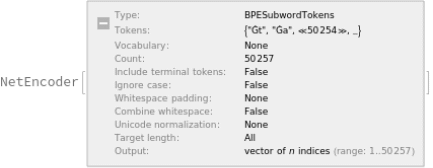The encoder produces an integer index for each subword token that corresponds to the position in the vocabulary:

 In:=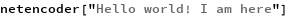Out=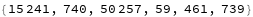Each subword token is also assigned a positional index:

 In:=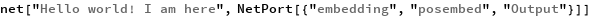Out=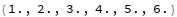A lookup is done to map these indices to numeric vectors of size 768:

 In:=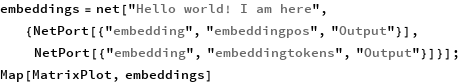Out=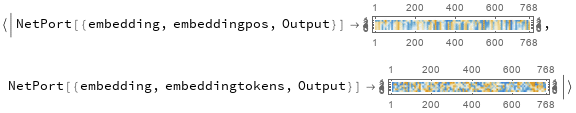For each subword token, these two embeddings are combined by summing elements with ThreadingLayer:

 In:=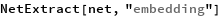Out=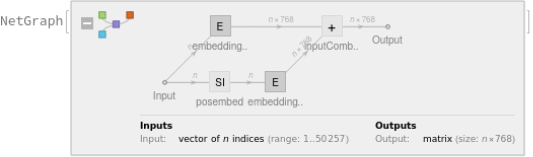The transformer architecture then processes the vectors using 12 structurally identical self-attention blocks stacked in a chain:

 In:=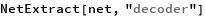Out=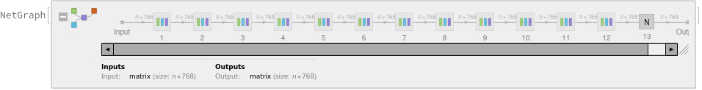The key part of these blocks is the attention module comprising of 12 parallel self-attention transformations, also called “attention heads”:

 In:=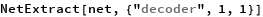Out=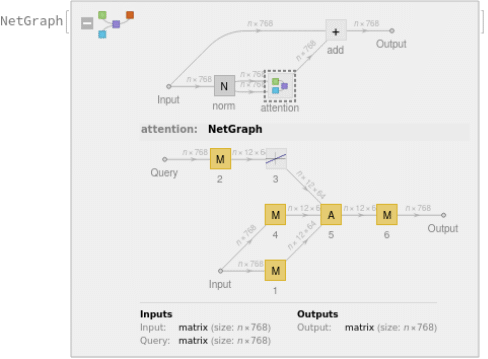Attention is done with causal masking, which means that the embedding of a given subword token depends on the previous subword tokens and not on the next ones. This is a prerequisite to be able to generate text with the language model. The following figures compare causal attention to other forms of connectivity between input tokens: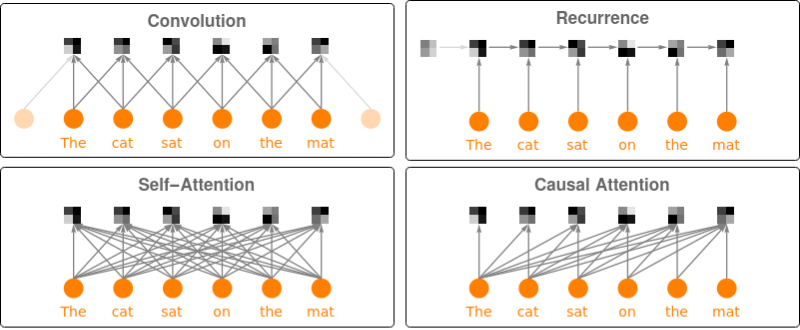### Language modeling

Retrieve the language model by specifying the "Task" parameter:

 In:=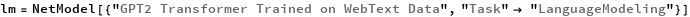Out=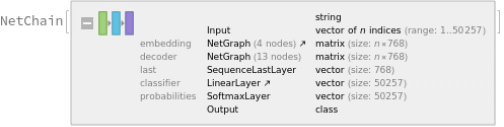Predict the next word in a given sequence:

 In:=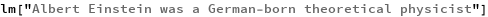Out=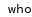Obtain the top 15 probabilities:

 In:=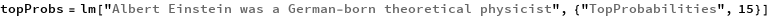Out=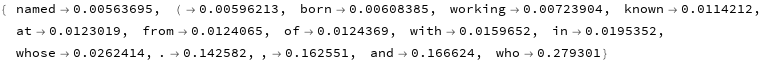Plot the top 15 probabilities:

 In:=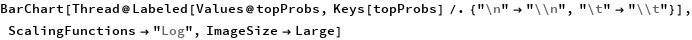Out=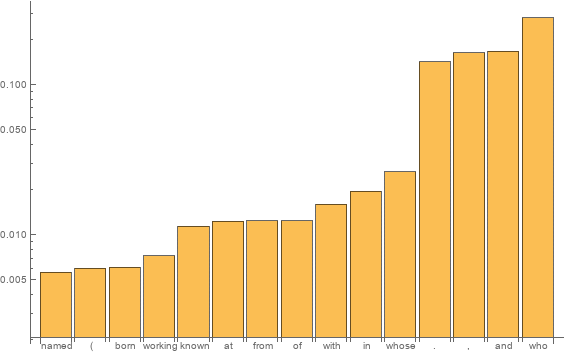### Text generation

Define a function to predict the next token:

 In:=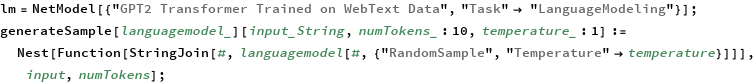Generate the next 20 tokens by using it on a piece of text:

 In:=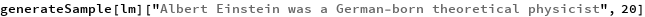Out=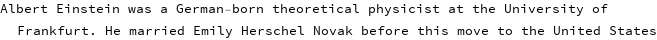The third optional argument is a “temperature” parameter that scales the input to the final softmax. A high temperature flattens the distribution from which tokens are sampled, increasing the probability of extracting less likely tokens:

 In:=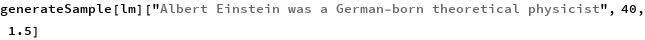Out=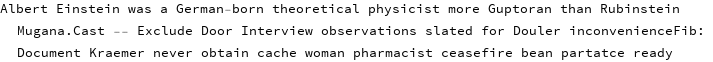Decreasing the temperature sharpens the peaks of the sampling distribution, further decreasing the probability of extracting less likely tokens:

 In:=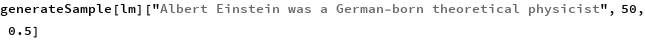Out=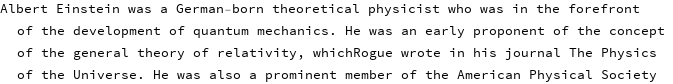Very high temperature settings are equivalent to random sampling:

 In:=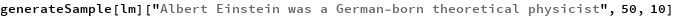Out=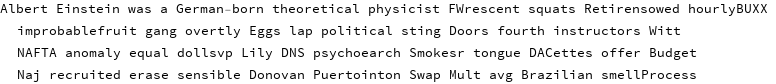Very low temperature settings are equivalent to always picking the character with maximum probability. It is typical for sampling to “get stuck in a loop”:

 In:=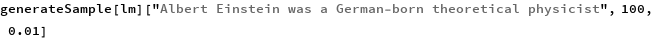Out=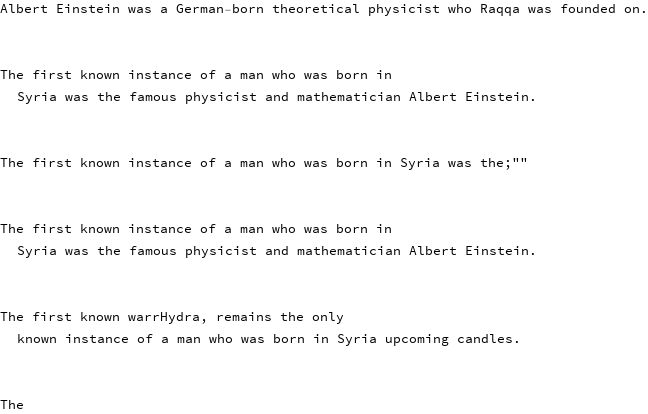### Sentence analogies

Define a sentence embedding that consists of the last subword embedding of GPT-2 (this choice is justified by the fact that GPT-2 is a forward causal model):

 In:=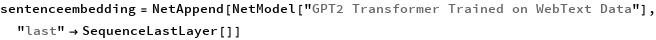Out=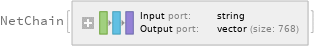Define some sentences in two broad categories for comparison:

 In:=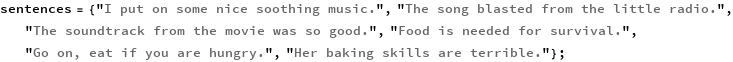Precompute the embeddings for a list of sentences:

 In:=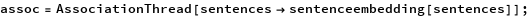Visualize the similarity between the sentences using the net as a feature extractor:

 In:=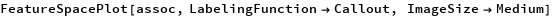Out=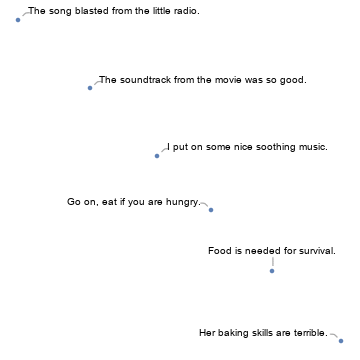### Train a classifier model with the subword embeddings

Get a text-processing dataset:

 In:=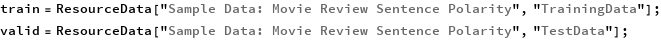View a random sample of the dataset:

 In:=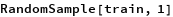Out=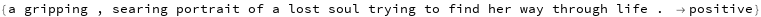Define a sentence embedding that consists of the last subword embedding of GPT-2 (this choice is justified by the fact that GPT-2 is a forward causal model):

 In:=Out=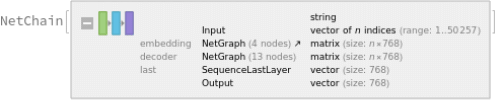Precompute the GPT-2 vectors for the training and the validation datasets (if available, GPU is recommended), using the last embedded vector as a representation of the entire text:

 In:=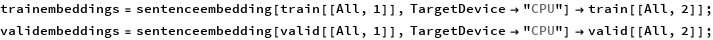Define a simple network for classification:

 In:=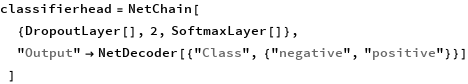Out=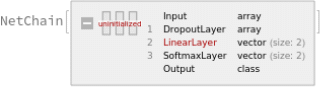Train the network on the precomputed GPT-2 vectors:

 In:=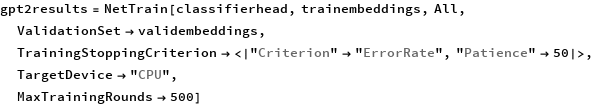Out=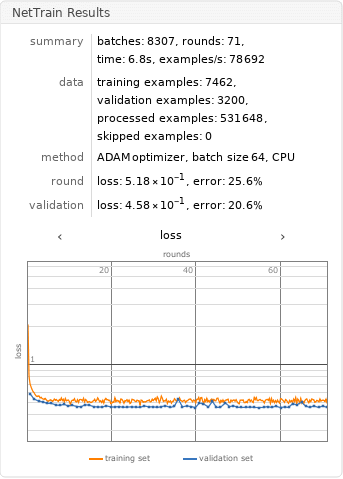Check the classification error rate on the validation data:

 In:=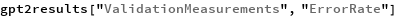Out=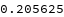Compare the results with the performance of a classifier trained on context-independent word embeddings. Precompute the GloVe vectors for the training and the validation datasets (if available, GPU is recommended):

 In:=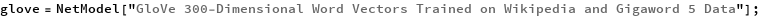In:=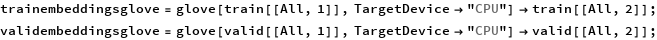Define a simple network for classification using a max-pooling strategy:

 In:=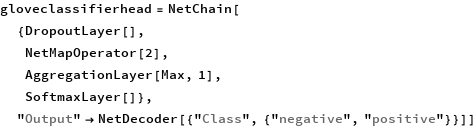Out=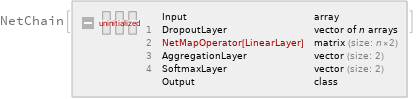Train the classifier on the precomputed GloVe vectors:

 In:=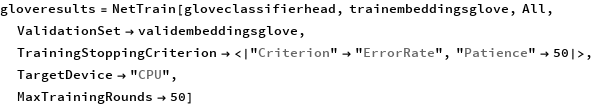Out=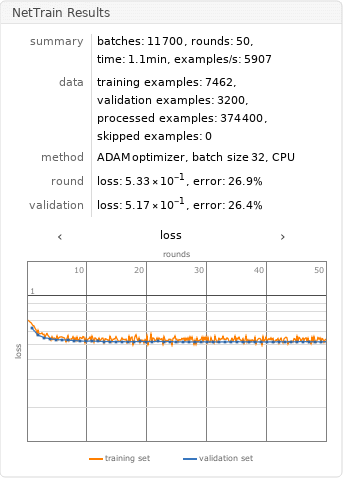Compare the results obtained with GPT-2 and with GloVe:

 In:=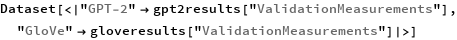Out=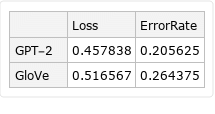### Net information

Inspect the number of parameters of all arrays in the net:

 In:=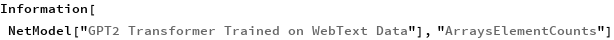Out=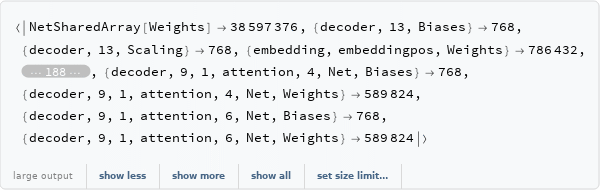Obtain the total number of parameters:

 In:=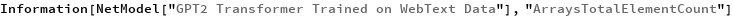Out=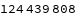Obtain the layer type counts:

 In:=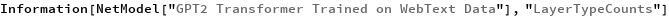Out=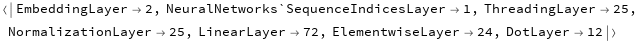Display the summary graphic:

 In:=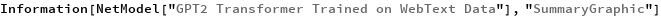Out=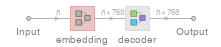### Export to MXNet

Export the net into a format that can be opened in MXNet:

 In:=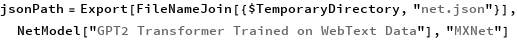Out=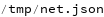Export also creates a net.params file containing parameters:

 In:=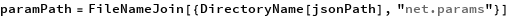Out=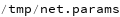Get the size of the parameter file:

 In:=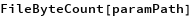Out=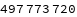## Requirements

Wolfram Language 12.1 (March 2020) or above